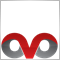# Problem Creating Class Objects[Deleted]
I am trying to create objects of a class that have a global scope. However when I try to assign the instances of the class to the variables (the pf array), I am receiving an error "'=' - object required". What am I doing wrong?
```//+------------------------------------------------------------------+
//| Classes                                                          |
//+------------------------------------------------------------------+
class SampleClass
{
public:
// Variables
int Length;
double ds[];

// Constructors
SampleClass(void){};
SampleClass(int length, int bufferNum)
{
Length=length;
SetIndexBuffer(bufferNum,ds);
};

void Calculate()
{
int bar = Bars-IndicatorCounted();
if (bar<Length)
return;

double var0, var1;
double sum = 0, sumif = 0;
for (int x=0;x<Length;x++)
{
double p1 = Close[x];
double p2 = Close[x+1];

sum+=p1*p1;
if (p1>p2)
sumif+=p1*p2;
}

var0=sumif;
var1=sum;

if (var1!=0)
ds[bar]=100*var0/var1;
else
ds[bar]=0;
};
};

SampleClass pf;

//+------------------------------------------------------------------+
//| Custom indicator initialization function                         |
//+------------------------------------------------------------------+
int init()
{
//---- indicators
IndicatorBuffers(1);
SetIndexStyle(0,DRAW_LINE);
SetIndexBuffer(0,indBuffer);

pf = new SampleClass(9,1);
pf = new SampleClass(19,2);
pf = new SampleClass(33,3);

//----
return(0);
}```3118

tymoore510:
I am trying to create objects of a class that have a global scope. However when I try to assign the instances of the class to the variables (the pf array), I am receiving an error "'=' - object required". What am I doing wrong?

The new operator returns a descriptor (a "dynamic pointer" in their terminology), so the declaration must be SampleClass* pf;

In that case you do not need the default constructor either.[Deleted]
Ovo:

The new operator returns a descriptor (a "dynamic pointer" in their terminology), so the declaration must be SampleClass* pf;

In that case you do not need the default constructor either.

That did the trick. Thank you very much for your assistance!